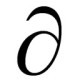## A nearly ubiquitous form of fallacious reasoning

by

How should one prove that two algebraic expressions are identically equal to one another?

Many people first learning to do proofs are tempted to start with the identity they seek to prove, then use various algebra (or trig or logarithmic or…) identities to manipulate both sides of the equation, seeking to reduce the equation to an easily-recognized identity.

But of course starting from$A = B$ and showing that this eventually implies that$1=1$ (or some other true equation) does not constitute a valid proof that$A=B$.

One of my favorite (simple) examples:$-3 \stackrel{?}{=} 3$$(-3)^2 \stackrel{?}{=} 3^2$$9=9$

Thus (the reasoning goes),$-3 = 3$, which is a fabulous result with many wonderful applications in the world of personal finance.

The problem is that each of the inferences being drawn is an if-then inference, for which knowing the consequent is true (9=9) in fact tells us nothing about the truth of the antecedent (-3=3). This form of argument can only be valid if every step is reversible — in effect if each step in the proof involves an if-and-only-if inference. But since most people learning proof are not particularly savvy at bearing this distinction in mind, good pedagogy suggests we completely avoid proving$A=B$ by reducing it to$1=1$. (Instead, one works from one side the equation to the other, applying identities to show that$A = A' = A'' = A'''=\cdots=B$.) [There are other ways to show that two expressions are equal, but this is the one most commonly relevant to elementary algebraic, trigonometric, and logarithmic identities.]

These issues hit home for me today when I read the March 2008 issue of Student Math Notes, published by the National Council of Teachers of Mathematics as an insert in the NCTM News Bulletin. This month’s issue focuses on the Pythagorean Theorem, and has a student worksheet exploring properties of right triangles.

Toward the end of the worksheet the students are told that if$m>n$ are whole numbers, then$m^2-n^2, 2mn, m^2+n^2$ will be a Pythagorean triple. The worksheet’s proof of this fact is to start with$(m^2-n^2)^2 + (2mn)^2 = (m^2+n^2)^2$

perform several algebraic operations on both sides of the equation, and reduce the given equation to the identity$m^4+2m^2n^2+n^4 = m^4+2m^2n^2+n^4$

“Since both sides are equal, it follows that$a^2+b^2=c^2$ and the three numbers form a Pythagorean Triple”, the authors conclude.

Technically, I guess their proof is okay, because in fact each step in the derivation (which I’ve omitted here) is in fact reversible. But the concluding sentence is dangerous and highly misleading, and I must say I am rather dismayed by it all.

A better proof would be to start with$(m^2-n^2)^2 + (2mn)^2$, note that that is equal to$(m^4 - 2m^2n^2 + n^4) + 4m^2n^2$, which simplifies to$m^4+2m^2n^2+n^4$, which by inspection is$(m^2+n^2)^2$.

What we lose in simplicity we gain in validity.

### 5 Responses to “A nearly ubiquitous form of fallacious reasoning”

1.Batman Says:

Or, as one of my professors put it, “It is good to be clever, but better to be correct.”

2.Ξ Says:

I’m not sure that the answer is to completely avoid it, but rather to emphasize the “if and only if” nature of the argument within the proof. (Although I admit, even though I talk about that necessity to all my classes and take off points if it’s left off, only about one such proof in fifteen “proofs” of this nature actually uses “if and only if” or explicitly points out that each step is reversible. It really is ingrained in many students that this type of argument is just fine as is.)

3.infinity Says:

I always hated seeing this correction on my homeworks/tests, but understood why it was there and when I was grading the other day, I wrote this correction down. It’s almost like you turn into your professors just like you turn into your parents…

4.A=B implies that 1=1, therefore… « 360 Says:

[…] implies that 1=1, therefore… By TwoPi I’ve ranted in the past about the fallacy of trying to prove an identity by starting with the equation itself, then […]

5.Don Moskowitz Says:

Unfortunately, the wording here is itself misleading and promotes a misconception. In fact, by noting why it is misleading, I believe the diagnosis can be simplified. In any event, assumptions are not per se antecedents, though any assumption may be used as one. To assume A=B would be to make it the case that any conditional with “A=B” as an antecedent would be true, under that assumption, were its consequent alone to follow from A=B. But that is only to say that any such conditional statement would follow from A=B, as opposed to the other away around. To be clear, that is the lesson meant to be drawn from the analysis provided. The problem with the analysis, however, is not that its conclusion is incorrect, but with the use of the phrase “starting with”. Its use suggests that on its heels one would find mention of an assumption, in this case “A=B”. But, of course, “A=B” does follow from the assumption “A=B”. It would not follow, however, if it were possible to correctly read the phrase as preceding, not the mention of an assumption, but of a conditional, with “A=B” as its antecedent. That is, in fact, the reading it is given in the analysis. By noting the absence of constraint placed on the consequent, such a reading serves as an invitation for the student to derive the wrong lesson: that the issue has to do with the fact that the consequent might be false. The point missed would be, then, that even with a true consequent, the problem remains. Since the key idea is that it is important to distinguish between assumptions and antecedents, explaining the problem by using a phrase that invites conflation would allow the problem to interfere with grasping it.